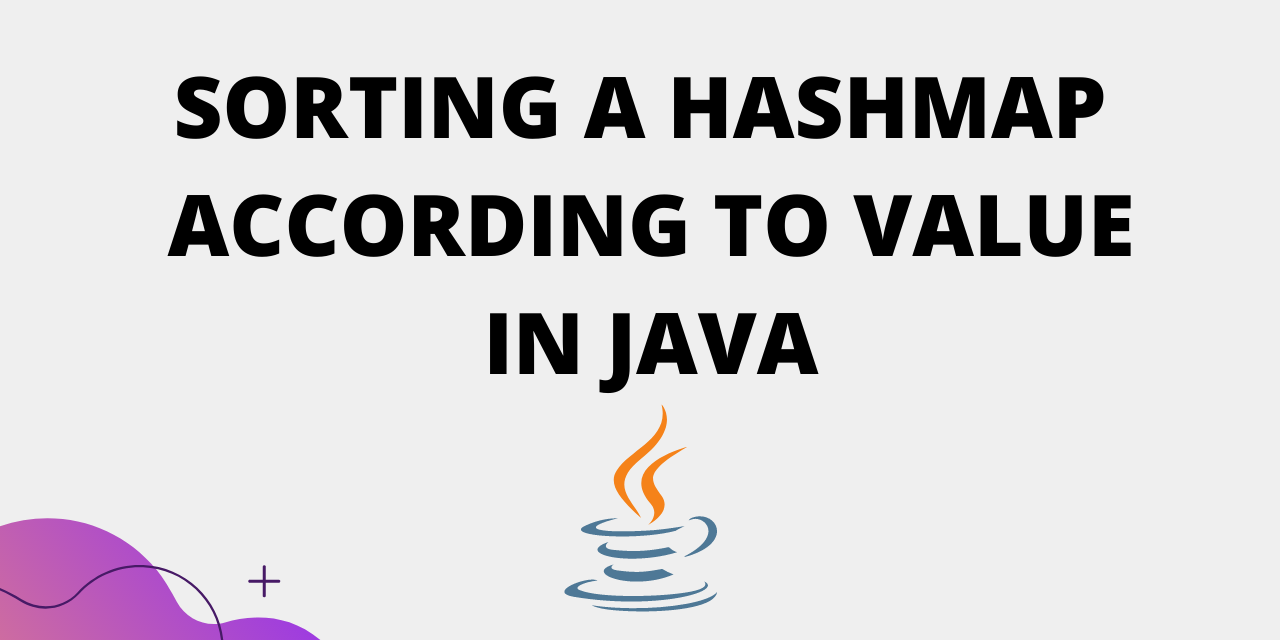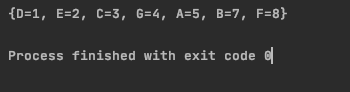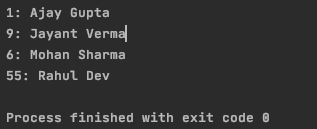# How to Sort a HashMap by Value in Java?

Published on August 3, 2022By Jayant VermaWhile we believe that this content benefits our community, we have not yet thoroughly reviewed it. If you have any suggestions for improvements, please let us know by clicking the “report an issue“ button at the bottom of the tutorial.

HashMap in java provides quick lookups. They store items in “key, value” pairs. To get a value from the HashMap, we use the key corresponding to that entry.

HashMaps are a good method for implementing Dictionaries and directories.

Key and Value can be of different types (eg - String, Integer).

We can sort the entries in a HashMap according to keys as well as values.

In this tutorial we will sort the HashMap according to value.

The basic strategy is to get the values from the HashMap in a list and sort the list. Here if the data type of Value is String, then we sort the list using a comparator. To learn more about comparator, read this tutorial.

Once we have the list of values in a sorted manner, we build the HashMap again based on this new list.

Let’s look at the code.

## Sorting HashMap by Value Simple Example

We first get the String values in a list. Then we sort the list.

To sort the String values in the list we use a comparator. This comparator sorts the list of values alphabetically.

`````` Collections.sort(list, new Comparator<String>() {
public int compare(String str, String str1) {
return (str).compareTo(str1);
}
});
``````

Once, we have sorted the list, we build the HashMap based on this sorted list.

The complete code is as follows :

``````package com.journaldev.collections;

import java.util.ArrayList;
import java.util.Collections;
import java.util.Comparator;
import java.util.HashMap;
import java.util.Map;
import java.util.Map.Entry;

public class Main {
public static void main(String[] args) {
HashMap<String, String> map = new HashMap<>();
ArrayList<String> list = new ArrayList<>();
map.put("2", "B");
map.put("8", "A");
map.put("4", "D");
map.put("7", "F");
map.put("6", "W");
map.put("19", "J");
map.put("1", "Z");
for (Map.Entry<String, String> entry : map.entrySet()) {
}
Collections.sort(list, new Comparator<String>() {
public int compare(String str, String str1) {
return (str).compareTo(str1);
}
});
for (String str : list) {
for (Entry<String, String> entry : map.entrySet()) {
if (entry.getValue().equals(str)) {
sortedMap.put(entry.getKey(), str);
}
}
}
System.out.println(sortedMap);
}
}

``````

Output :

``````{8=A, 5=B, 3=D, 7=F, 10=J, 2=W, 1=Z}
``````

HashMap entries are sorted according to String value.

## Another Example of Sorting HashMap by Value

If values in the HashMap are of type Integer, the code will be as follows :

``````package com.JournalDev;

import java.util.ArrayList;
import java.util.Collections;
import java.util.Comparator;
import java.util.HashMap;
import java.util.Map;
import java.util.Map.Entry;

public class Main {
public static void main(String[] args) {
HashMap<String, Integer> map = new HashMap<>();
ArrayList<Integer> list = new ArrayList<>();
map.put("A", 5);
map.put("B", 7);
map.put("C", 3);
map.put("D", 1);
map.put("E", 2);
map.put("F", 8);
map.put("G", 4);
for (Map.Entry<String, Integer> entry : map.entrySet()) {
}
Collections.sort(list);
for (int num : list) {
for (Entry<String, Integer> entry : map.entrySet()) {
if (entry.getValue().equals(num)) {
sortedMap.put(entry.getKey(), num);
}
}
}
System.out.println(sortedMap);
}
}

``````

Output :

``````{D=1, E=2, C=3, G=4, A=5, B=7, F=8}
``````Here HashMap values are sorted according to Integer values.

## Sorting the HashMap using a custom comparator

If you notice the above examples, the Value objects implement the Comparator interface. Let’s look at an example where our value is a custom object.

We can also create a custom comparator to sort the hash map according to values. This is useful when your value is a custom object.

Let’s take an example where value is a class called ‘Name’. This class has two parameters, firstName and lastName.

The code for class Name is :

``````package com.JournalDev;

public class Name {
String firstName;
String lastName;
Name(String a, String b){
firstName=a;
lastName=b;

}
public String getFirstName() {
return firstName;
}

}

``````

The custom comparator will look like :

``````Comparator<Name> byName = (Name obj1, Name obj2) -> obj1.getFirstName().compareTo(obj2.getFirstName());
``````

We are sorting the names according to firstName, we can also use lastName to sort. Rather than using a list to get values from the map, we’ll be using LinkedHashMap to create the sorted hashmap directly.

The complete code is :

``````public static void main(String[] args) {
HashMap<Integer, Name> hmap = new HashMap<Integer, Name>();
Name name1 = new Name("Jayant", "Verma");
Name name2 = new Name("Ajay", "Gupta");
Name name3 = new Name("Mohan", "Sharma");
Name name4 = new Name("Rahul", "Dev");

hmap.put(9, name1);
hmap.put(1, name2);
hmap.put(6, name3);
hmap.put(55, name4);

Comparator<Name> byName = (Name obj1, Name obj2) -> obj1.getFirstName().compareTo(obj2.getFirstName());

.sorted(Map.Entry.<Integer, Name>comparingByValue(byName))
.collect(Collectors.toMap(Map.Entry::getKey, Map.Entry::getValue, (e1, e2) -> e1, LinkedHashMap::new));

//printing the sorted hashmap
Set set = sortedMap.entrySet();
Iterator iterator = set.iterator();
while (iterator.hasNext()) {
Map.Entry me2 = (Map.Entry) iterator.next();
System.out.print(me2.getKey() + ": ");
System.out.println(hmap.get(me2.getKey()).firstName + " "+hmap.get(me2.getKey()).lastName );

}
}
``````

Output :

``````1: Ajay Gupta
9: Jayant Verma
6: Mohan Sharma
55: Rahul Dev
``````## Conclusion

This tutorial covered sorting of HashMap according to Value. Sorting for String values differs from Integer values. String values require a comparator for sorting. Whereas, Integer values are directly sorted using Collection.sort().

Thanks for learning with the DigitalOcean Community. Check out our offerings for compute, storage, networking, and managed databases.Jayant Verma

author

#### Still looking for an answer?

Ask a questionSearch for more help

Click below to sign up and get \$200 of credit to try our products over 60 days!

### Popular Topics##### Hollie's Hub for Good

Working on improving health and education, reducing inequality, and spurring economic growth? We’d like to help.##### Become a contributor

You get paid; we donate to tech nonprofits.

##### Welcome to the developer cloud

DigitalOcean makes it simple to launch in the cloud and scale up as you grow – whether you’re running one virtual machine or ten thousand.© 2023 DigitalOcean, LLC.